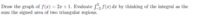# Integral of Two Triangles

#### Hckyplayer8

##### Full MemberI sketched the function which is a diagonal line. I placed [-2,2] as my bounds and found f(-2) = -3 and f(2) = 5. They served as the height of my two triangles.

My next task was to find the x intercept of the function as that designated the change from negative area to positive area. I found the x intercept to be -1/2. Then it was just a matter of finding the x coord distance between -2 and -1/2, as well as -1/2 and 2 to serve as the base of the triangles.

I labeled the triangle with positive area as A1 and the triangle with negative area as A2.

Thus for A1 = [2.5 * 5] / 2 = 6.25 and for A2 [3*1.5] / 2 = 2.25

Then for the final answer A1 - A2 = 6.25 - 2.25 = 4

Does this look reasonable?

•Subhotosh Khan

#### Dr.Peterson

##### Elite Member
Yes, everything looks just right.

•Hckyplayer8

#### Hckyplayer8

##### Full Member
Sweet! Appreciate it.